## Inscribed circle in a triangle

June 12, 2023 Uncategorized

## Motivation

In Jim Smith’s recent Handling Rejection! Using Geometric Algebra to Find the Incircle of a Triangle video, he uses an area argument to find the center point of a circle inscribed in a triangle, as illustrated in fig. 1.

In the video, Jim mentioned that he first tried calculating the intersection of the bivectors, but didn’t like the form of the solution. I’m curious what aspect of that solution wasn’t desirable, since it is a pretty compact way to solve the system.

## Setup.

A very convenient way to describe a triangle is with a pair of vectors for two of the edges, say $$\Ba, \Bb$$, where the third edge is $$\Bc = \Ba -\Bb$$. In Jim’s problem, he started with the scalar lengths of all the edges $$a, b, c$$. The two representations are interchangable. We can set
\begin{equation}\label{eqn:triangleInscribedCircle:n}\
\begin{aligned}
\Ba &= a\Be_1 \\
\Bb &= b \Be_1 e^{i\theta_c},
\end{aligned}
\end{equation}
where $$i = \Be_1 \Be_2$$, and $$\theta_c$$ is the angle opposite edge $$\Bc$$, which can be found from the cosine law
\begin{equation}\label{eqn:triangleInscribedCircle:20}
\Bc^2 = \lr{ \Ba – \Bb }^2 = \Ba^2 + \Bb^2 – 2 \Ba \cdot \Bb = a^2 + b^2 – 2 a b \cos\theta_c,
\end{equation}
or
\begin{equation}\label{eqn:triangleInscribedCircle:40}
\theta_c = \cos^{-1} \lr{ \frac{a^2 + b^2 – c^2}{2 a b} }.
\end{equation}

## The center point.

The points on our bisectors are
\begin{equation}\label{eqn:triangleInscribedCircle:60}
\begin{aligned}
r_c(t) &= t \lr{ \mathbf{\hat{a}} + \mathbf{\hat{b}} } \\
r_b(t) &= \Ba + t \lr{ \mathbf{\hat{a}} + \mathbf{\hat{c}} } \\
r_a(t) &= \Bb + t \lr{ \mathbf{\hat{c}} – \mathbf{\hat{b}} }.
\end{aligned}
\end{equation}
We can find the intersection of any two of these to find the center point of the circle. For example, we seek solutions of
\begin{equation}\label{eqn:triangleInscribedCircle:80}
u \lr{ \mathbf{\hat{a}} + \mathbf{\hat{b}} } = \Ba + v \lr{ \mathbf{\hat{a}} + \mathbf{\hat{c}} }.
\end{equation}
We can wedge this with either of the $$u, v$$ vector factors, to eliminate one of the scalars in this equation. Seeking $$u$$, we wedge with $$\mathbf{\hat{a}} + \mathbf{\hat{c}}$$, for
\begin{equation}\label{eqn:triangleInscribedCircle:100}
u \lr{ \mathbf{\hat{a}} + \mathbf{\hat{c}} } \wedge \lr{ \mathbf{\hat{a}} + \mathbf{\hat{b}} } = \lr{ \mathbf{\hat{a}} + \mathbf{\hat{c}} } \wedge \Ba = \mathbf{\hat{c}} \wedge \Ba.
\end{equation}
so
\begin{equation}\label{eqn:triangleInscribedCircle:120}
\Bz = \lr{ \mathbf{\hat{a}} + \mathbf{\hat{b}} } \lr{ \lr{ \mathbf{\hat{c}} \wedge \Ba } \cdot \inv{ \lr{ \mathbf{\hat{a}} + \mathbf{\hat{c}} } \wedge \lr{ \mathbf{\hat{a}} + \mathbf{\hat{b}} } } }.
\end{equation}
Having found the center, we can calculate the radius that touches the $$a$$ edge, which is just
\begin{equation}\label{eqn:triangleInscribedCircle:140}
\mathrm{Rej}_{\Ba}(\Bz) = \lr{ \Bz \wedge \mathbf{\hat{a}} } \cdot \mathbf{\hat{a}}.
\end{equation}
\begin{equation}\label{eqn:triangleInscribedCircle:160}
r = \Norm{ \Bz \wedge \mathbf{\hat{a}} }.
\end{equation}

## Calculation.

Using a Mathematica Manipulate, I plotted this solution, employing a duality transformation to calculate the bivectors using cross products (avoiding any GA package dependencies.)

For the radius, we need only:
\begin{equation}\label{eqn:triangleInscribedCircle:200}
r = \Norm{ \Bz \cross \mathbf{\hat{a}} }.
\end{equation}

For the intersection, we can use:
\begin{equation}\label{eqn:triangleInscribedCircle:180}
\Bz = \lr{ \mathbf{\hat{a}} + \mathbf{\hat{b}} } \lr{ \lr{ \mathbf{\hat{c}} \cross \Ba } \cdot \inv{ \lr{ \mathbf{\hat{a}} + \mathbf{\hat{c}} } \cross \lr{ \mathbf{\hat{a}} + \mathbf{\hat{b}} } } }.
\end{equation}

In retrospect, just using a ratio of the determinants of the coordinates would have been a direct representation of the ratio of the wedge products, so I could have done that instead to translate the results from GA to Mathematica (since this is a planar problem.)  The wedge products could have also been encoding using complex numbers (in the newest version of the notebook, I’ve done that.)

Of course, using Mathematica, we could have just used it’s more general $$\textrm{Solve}$$ function, and need not grow our own wedge product based implementation.

## Spoiling Karl: a productive day of fishing for cat6 flavoured wall trout.

November 13, 2022 Uncategorized

When we moved into the new house, we said to Karl that we could probably run ethernet wiring up to his room from my office where the router lives.  He’s been anxious for us to try that project, to say the least.

Today was the day to try that.  I started by cutting a very large hole in his wall to see into the big triangular roof space.  I wanted a hole that was big enough that we could potentially reach into the back, since I was pretty sure that I could get a line up in that location.  Here’s that hole with the fishing wire poking out: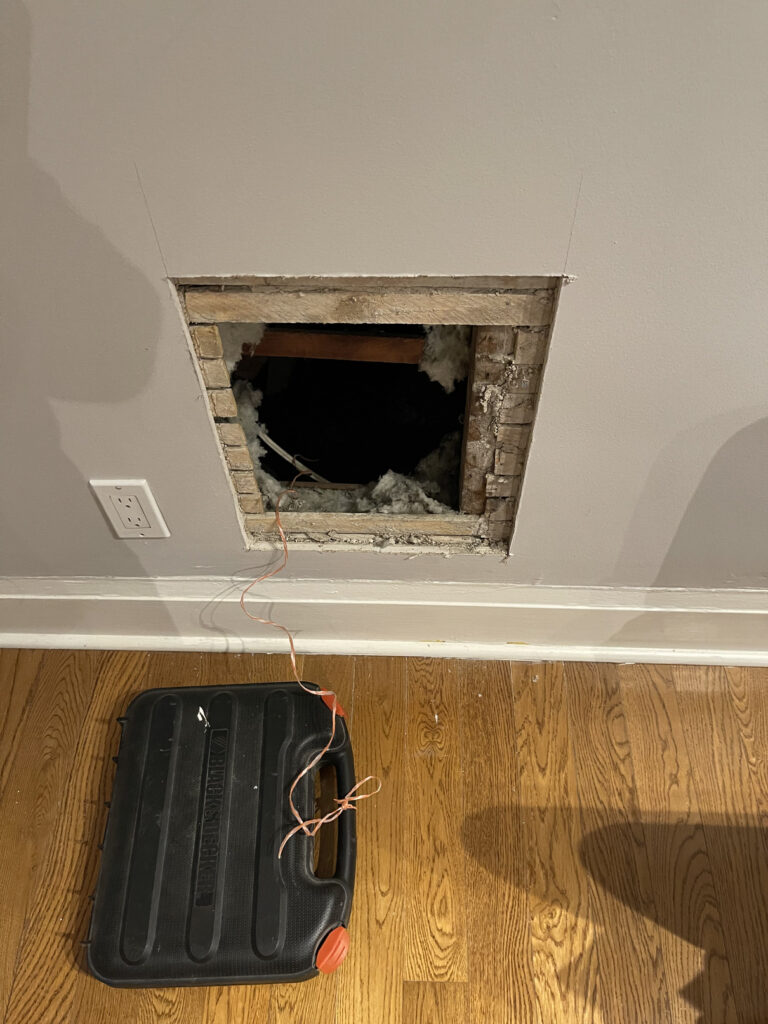The house is old, and the walls have been finished with drywall over plaster and lathe.

In my office I had to cut two holes, one near the ceiling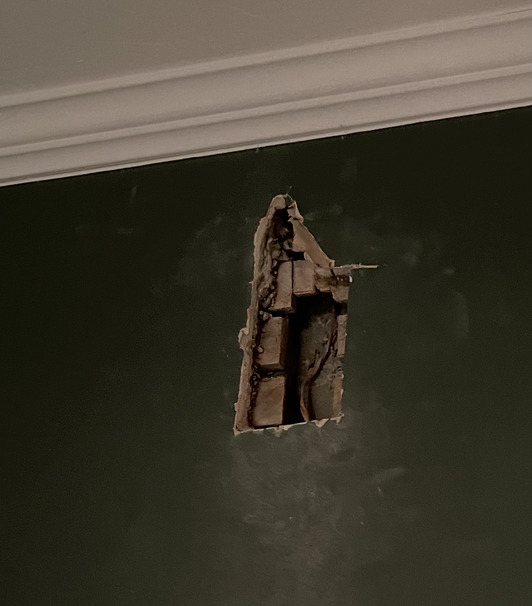I cut a nice little rectangle with the intention of using my 4.5′ flexible 3/4″ drill bit between the lathe and the brick, but changed my strategy once I saw the hole.  We’ve got the following layers in this space:

• drywall (1/2″)
• plaster (~1/2″)
• lathe (~1/2″)
• 5/8-3/4″ gap (no insulation)
• brick.

I was worried that trying to jam my drill bit into the space between the lathe and the brick would wreck it, since the space is so small.  Instead I opted to try to drill upwards through the plaster layer, into the lathe, and then up through the ceiling.  This wasn’t the easiest path to drill and the bit wandered significantly, but I did get the hole made, and fished my pulling line up.  With Karl’s help, we got the pulling line out of our big hole.  I was then ready to cut my second hole, and try to get the line down from the ceiling area to closer to the floor, where I’ll put the outlet and the ethernet jacks.  Here’s that hole with the pulling line fished through: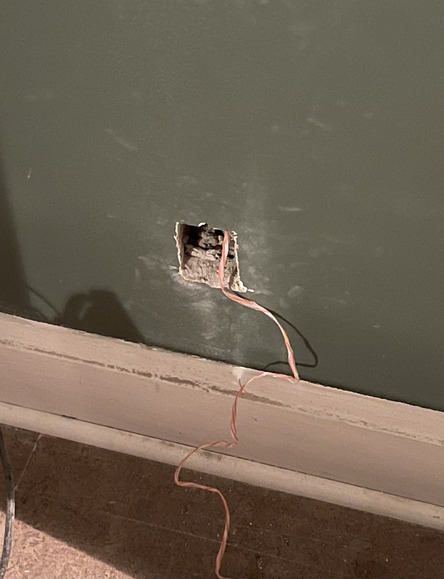I chose to run 4 lines.  Two for Karl’s room, and two for the back room.  The two lines for the back room, have just been left in the crawl space.  I tossed them in a bit, so that if we open up the wall in that room, it should be possible to crawl in from the other side and grab them.  We are thinking that it would be good to open up that triangular dead space and build in some sort of integrated storage solution — at which point we could also run ethernet to that room.  Even if we don’t use it, it’s easy to leave it there for the future at this point when the walls are opened up.

Here’s Karl’s room temporarily closed up with one jack connected: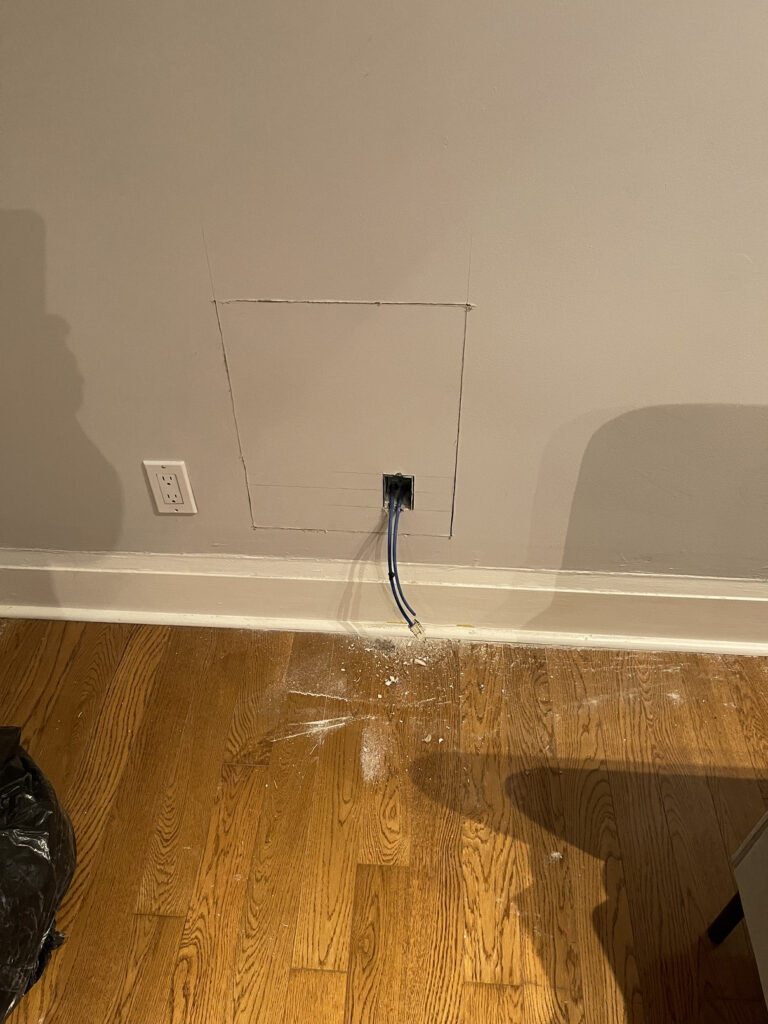The end result from Karl’s point of view is the following speed test: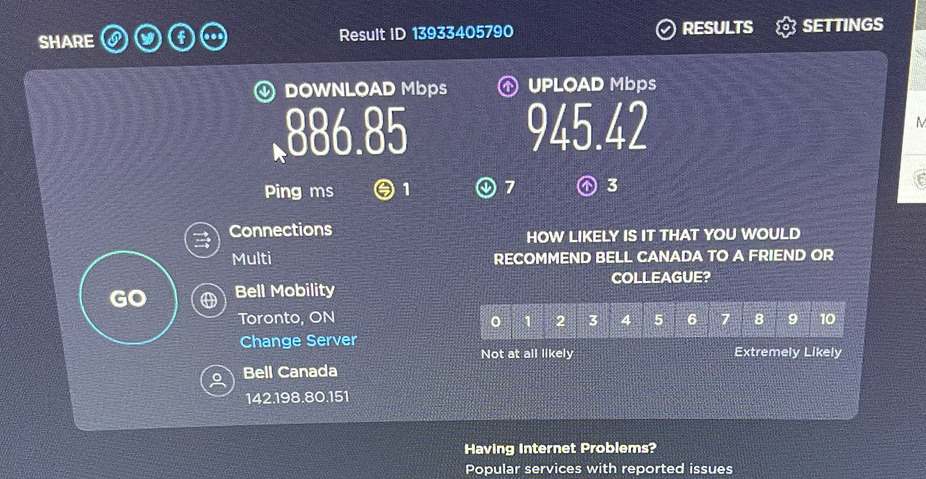He was very happy with this result, about 5x faster than his wifi speed (which in turn, is also way better than his wifi speed at the old house.)

There is still more to do for this little project:

• reinsert the insulation in the big hole, and anchor the drywall in place, and the mud and sanding to patch things up.
• patch up things in my office and repaint that little wall (hopefully, I can get away with just that single wall.)
• connect jacks for the second wire, and put on covers (on order.)
• (maybe) connect jacks for the “future expansion” wires for the back room.  I may just leave those in the wall for now (but have ordered a 4-port jack plate.)

## A bitcoin ransom entrepreneur

March 25, 2021 Uncategorized , ,

I call the bluff. Let’s see the video!

Hello!

Unfortunately, I have some bad news for you.
Several months ago, I got access to the device you are using to browse the internet.
Since that time, I have been monitoring your internet activity.

Being a regular visitor of adult websites, I can confirm that it is you who is responsible for this.

I've uploaded a Trojan horse on the driver basis that updates its signature several times per day, to make it impossible for antivirus to detect it. Additionally, it gives me access to your camera and microphone.
Moreover, I have backed-up all the data, including photos, social media, chats and contacts.
Just recently, I came up with an awesome idea to create the video where you cum in one part of the screen, while the video was simultaneously playing on another screen. That was fun!

Rest assured that I can easily send this video to all your contacts with a few clicks, and I assume that you would like to prevent this scenario. )

With that in mind, here is my proposal:
Transfer the amount equivalent to 1350 USD to my Bitcoin wallet, and I will forget about the entire thing. I will also delete all data and videos permanently.

In my opinion, this is a somewhat modest price for my work.
You can figure out how to purchase Bitcoins using search engines like Google or Bing, seeing that it's not very difficult.

My Bitcoin wallet (BTC):
1Lg6nE6zFaMZHiPTFR9nSwuAL6hzeegToC

You have 48 hours to reply and you should also bear the following in mind:

It makes no sense to reply me - the address has been generated automatically
It makes no sense to complain either, since the letter along with my Bitcoin wallet cannot be tracked.
Everything has been orchestrated precisely.

If I ever detect that you mentioned anything about this letter to anyone - the video will be immediately shared, and your contacts will be the first to receive it. Following that, the video will be posted on the web!

P.S. The time will start once you open this letter. (This program has a built-in timer and special pixel ID - [№78853473])
Good luck and take it easy! It was just bad luck, next time please be careful.


## Hardcover edition of Geometric Algebra for Electrical Engineers.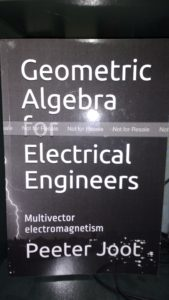I was invited to Kindle Direct Publishing‘s hardcover beta program, and have made my geometric algebra book available in black and white hardcover.

As always, the PDF, leanpub edition, and latex sources are also available.

I thought that it was too confusing to have color and black-and-white editions of the book (color has a significantly higher printing cost), so I have unpublished the color editions of the book (softcover, and hardcover). There is one copy of the color edition left, and once that is sold, it will show as out of print.

## New version of classical mechanics notes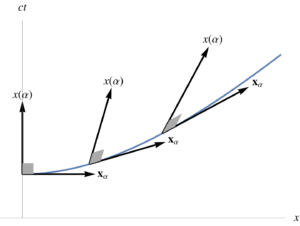I’ve posted a new version of my classical mechanics notes compilation.  This version is not yet live on amazon, but you shouldn’t buy a copy of this “book” anyways, as it is horribly rough (if you want a copy, grab the free PDF instead.)  [I am going to buy a copy so that I can continue to edit a paper copy of it, but nobody else should.]

This version includes additional background material on Space Time Algebra (STA), i.e. the geometric algebra name for the Dirac/Clifford-algebra in 3+1 dimensions.  In particular, I’ve added material on reciprocal frames, the gradient and vector derivatives, line and surface integrals and the fundamental theorem for both.  Some of the integration theory content might make sense to move to a different book, but I’ll keep it with the rest of these STA notes for now.